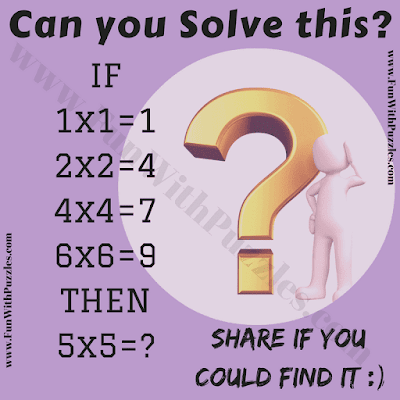This Mathematics Brain Teaser is challenge for school going teens. In this Brain Crackling Challenge you are given some number equations. These numbers equations follows some logical pattern. Your challenge is to crack this hidden pattern and then solve the last equation to find the value of the missing number which replaces question mark. Lets see if you can solve this Brain Crackling Challenge?Can you solve this brain crackling challenge?

Answer of this "Brain Crackling Challenge in Mathematics", can be viewed by clicking on button. Please do give your best try before looking at the answer.

#### 1 comment:

Unknown said...

5 * 5= 7
you add the digits of the number squared
a^2= bc, which are added b+c